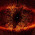## Friday, February 8, 2013

### Moving and Rotation Static Physijs Objects

‹prev | My Chain | next›

I ran into a bit of a problem with my Three.js / Physijs "puzzle" game yesterday. The static ramps that the player can move around a room to reach the prize can rotate or they can move:

But bad things happen when I try to both spin and move the ramps. Even if I spin the ramp a bit then move it, the ramp ends up getting repositioned and reoriented back where it started. These ramps are static Physijs meshes, which require some effort to move and rotate—mostly marking the updated position / rotation as "dirty" so that the physics simulator does not get confused.

To figure out what is going on, I follow the tried and true strategy of trying to repeat with a simple case. If it works in the simple case, then something I am doing in my complicated game code is causing the problem. If it fails in the simple case, then Physijs has a bug. So I build a simple Physijs mesh, and set it spinning even as the Physijs simulator does its thing:
``````  var mesh = new Physijs.ConvexMesh(
new THREE.CubeGeometry(10, 100, 10),
Physijs.createMaterial(
new THREE.MeshBasicMaterial({color:0x0000cc}), 0.2, 1.0
),
0
);

var dir = 1;
function animate() {
requestAnimationFrame(animate);

mesh.__dirtyRotation = true;
mesh.rotation.z = mesh.rotation.z + 0.01;

mesh.__dirtyPosition = true;
mesh.position.x = mesh.position.x + 0.5*dir;
mesh.position.y = mesh.position.y + 0.5*dir;

if (Math.abs(mesh.position.x) > 100) dir = -1 * dir;

scene.simulate(); // run physics

renderer.render(scene, camera);
}
animate();``````
(running example)

And it works. My "static" ramp (static by virtue of the zero argument in the constructor) moves around the screen and rotates as it does. So it is possible, which means that I am doing something wrong.

The fun part now is to either add stuff to the simple case until it gets complicated enough to fail in the same way or to simplify my game until it works in the same way. It is always easiest to start with the former. I start by moving the physics out of the `animate()` function into its own, less frequently processed, function:
``````  function gameStep() {
// mesh.rotation.z = mesh.rotation.z + 0.01;
mesh.rotation.set(0,0,mesh.rotation.z + 0.01);
mesh.__dirtyRotation = true;

mesh.position.x = mesh.position.x + 0.5*dir;
mesh.position.y = mesh.position.y + 0.5*dir;
mesh.__dirtyPosition = true;

if (Math.abs(mesh.position.x) > 100) dir = -1 * dir;

scene.simulate(); // run physics

// process the game logic at a target 60 FPS
setTimeout(gameStep, 1000/6);
}
gameStep();``````
And it still works.

Next, I try moving and rotating until I reach an arbitrary distance from the start part. Once I hit that mark, I stop the movement, but continue the rotation:
``````    // ...
if (mesh.position.x < 50) {
mesh.position.x = mesh.position.x + 0.5*dir;
mesh.position.y = mesh.position.y + 0.5*dir;
mesh.__dirtyPosition = true;
}
// ...``````
With that, I have finally complicated it enough to make it fail.

After the movement stops, the next rotation causes the ramp to jump back to the origin. It seems that once I have made a dirty Physijs movement, I have to remember to mark the position as dirty for all future movement or rotation. In other words, back in my game, I have to make the position and rotation as dirty—even if only changing one or the other:
``````  var ramp = {
startAt: function(location) {
this.mesh = new Physijs.ConvexMesh( /* ... */ );
// ...
},
var mesh = this.mesh;
mesh.position.x = mesh.position.x + event.x_diff;
mesh.position.y = mesh.position.y + event.y_diff;
mesh.__dirtyRotation = true;
mesh.__dirtyPosition = true;
});
},
var mesh = this.mesh;
if (!mesh.isActive) return;
if (event.keyCode != 114) return;
mesh.rotation.set(0,0,mesh.rotation.z + 0.1);
mesh.__dirtyRotation = true;
mesh.__dirtyPosition = true;
});
},
// ...
};``````
With that, I can rotate and move the ramps fast enough to trap my player on the top of the screen:

It seems odd that I need to mark the position as dirty to prevent a subsequent dirty rotation, but that's what happens with things are dirty—code gets messy.

Day #656

1.2.